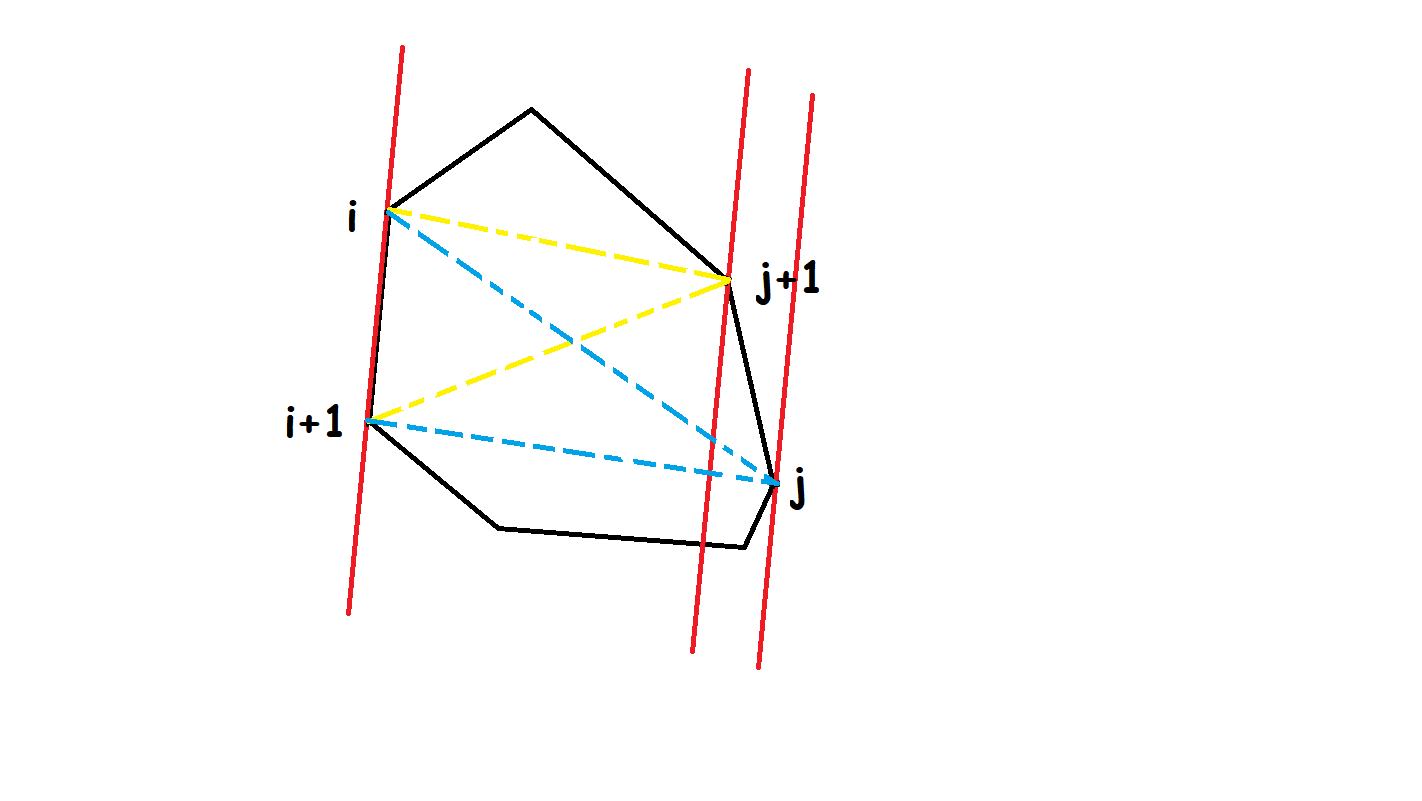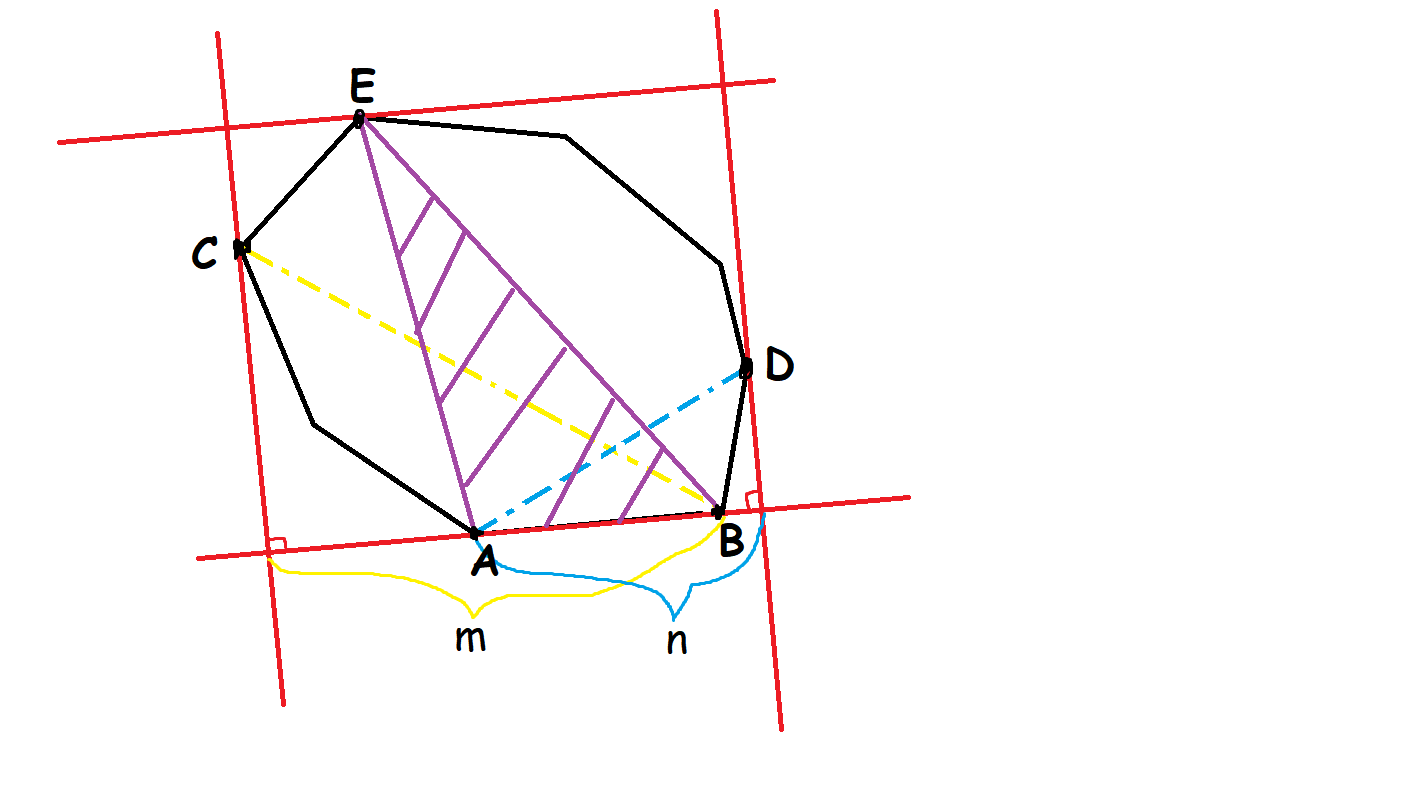# 旋转卡壳

## 求凸包直径

### 过程### 实现

  1 2 3 4 5 6 7 8 9 10 11 12 13 14 15 16 17 18 19 20 21 22 23 24 int sta[N], top; // 将凸包上的节点编号存在栈里，第一个和最后一个节点编号相同 bool is[N]; ll pf(ll x) { return x * x; } ll dis(int p, int q) { return pf(a[p].x - a[q].x) + pf(a[p].y - a[q].y); } ll sqr(int p, int q, int y) { return abs((a[q] - a[p]) * (a[y] - a[q])); } ll mx; void get_longest() { // 求凸包直径 int j = 3; if (top < 4) { mx = dis(sta, sta); return; } for (int i = 1; i <= top; ++i) { while (sqr(sta[i], sta[i + 1], sta[j]) <= sqr(sta[i], sta[i + 1], sta[j % top + 1])) j = j % top + 1; mx = max(mx, max(dis(sta[i + 1], sta[j]), dis(sta[i], sta[j]))); } } 
  1 2 3 4 5 6 7 8 9 10 11 12 13 14 15 16 17 sta = [] * N; top = 0 # 将凸包上的节点编号存在栈里，第一个和最后一个节点编号相同 def pf(x): return x * x def dis(p, q): return pf(a[p].x - a[q].x) + pf(a[p].y - a[q].y) def sqr(p, q, y): return abs((a[q] - a[p]) * (a[y] - a[q])) def get_longest(): # 求凸包直径 j = 3 if top < 4: mx = dis(sta, sta) return for i in range(1, top + 1): while sqr(sta[i], sta[i + 1], sta[j]) <=\ sqr(sta[i], sta[i + 1], sta[j % top + 1]): j = j % top + 1 mx = max(mx, max(dis(sta[i + 1], sta[j]), dis(sta[i], sta[j]))) 

## 求最小矩形覆盖

Luogu P3187 最小矩形覆盖

### 过程### 实现

  1 2 3 4 5 6 7 8 9 10 11 12 13 14 15 16 17 18 19 20 void get_biggest() { int j = 3, l = 2, r = 2; double t1, t2, t3, ans = 2e10; for (int i = 1; i <= top; ++i) { while (sqr(sta[i], sta[i + 1], sta[j]) <= sqr(sta[i], sta[i + 1], sta[j % top + 1])) j = j % top + 1; while (dot(sta[i + 1], sta[r % top + 1], sta[i]) >= dot(sta[i + 1], sta[r], sta[i])) r = r % top + 1; if (i == 1) l = r; while (dot(sta[i + 1], sta[l % top + 1], sta[i]) <= dot(sta[i + 1], sta[l], sta[i])) l = l % top + 1; t1 = sqr(sta[i], sta[i + 1], sta[j]); t2 = dot(sta[i + 1], sta[r], sta[i]) + dot(sta[i + 1], sta[l], sta[i]); t3 = dot(sta[i + 1], sta[i + 1], sta[i]); ans = min(ans, t1 * t2 / t3); } } 
  1 2 3 4 5 6 7 8 9 10 11 12 13 14 15 16 17 18 19 def get_biggest(): j = 3; l = 2; r = 2 ans = 2e10 for i in range(1, top + 1): while sqr(sta[i], sta[i + 1], sta[j]) <=\ sqr(sta[i], sta[i + 1], sta[j % top + 1]): j = j % top + 1 while dot(sta[i + 1], sta[r % top + 1], sta[i]) >=\ dot(sta[i + 1], sta[r], sta[i]): r = r % top + 1 if i == 1: l = r while dot(sta[i + 1], sta[l % top + 1], sta[i]) <=\ dot(sta[i + 1], sta[l], sta[i]): l = l % top + 1 t1 = sqr(sta[i], sta[i + 1], sta[j]) t2 = dot(sta[i + 1], sta[r], sta[i]) + dot(sta[i + 1], sta[l], sta[i]) t3 = dot(sta[i + 1], sta[i + 1], sta[i]) ans = min(ans, t1 * t2 / t3) 

## 参考资料与注释

1. Toussaint, Godfried T. (1983). "Solving geometric problems with the rotating calipers". Proc. MELECON '83, Athens. CiteSeerX 10.1.1.155.5671